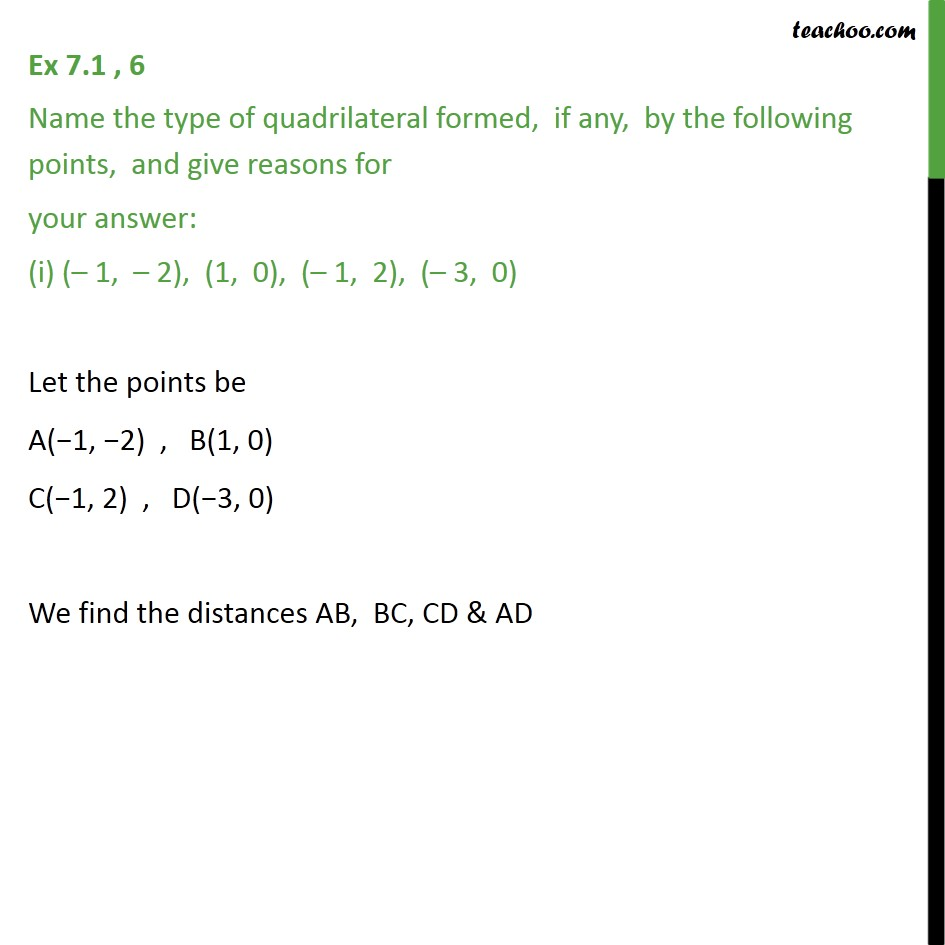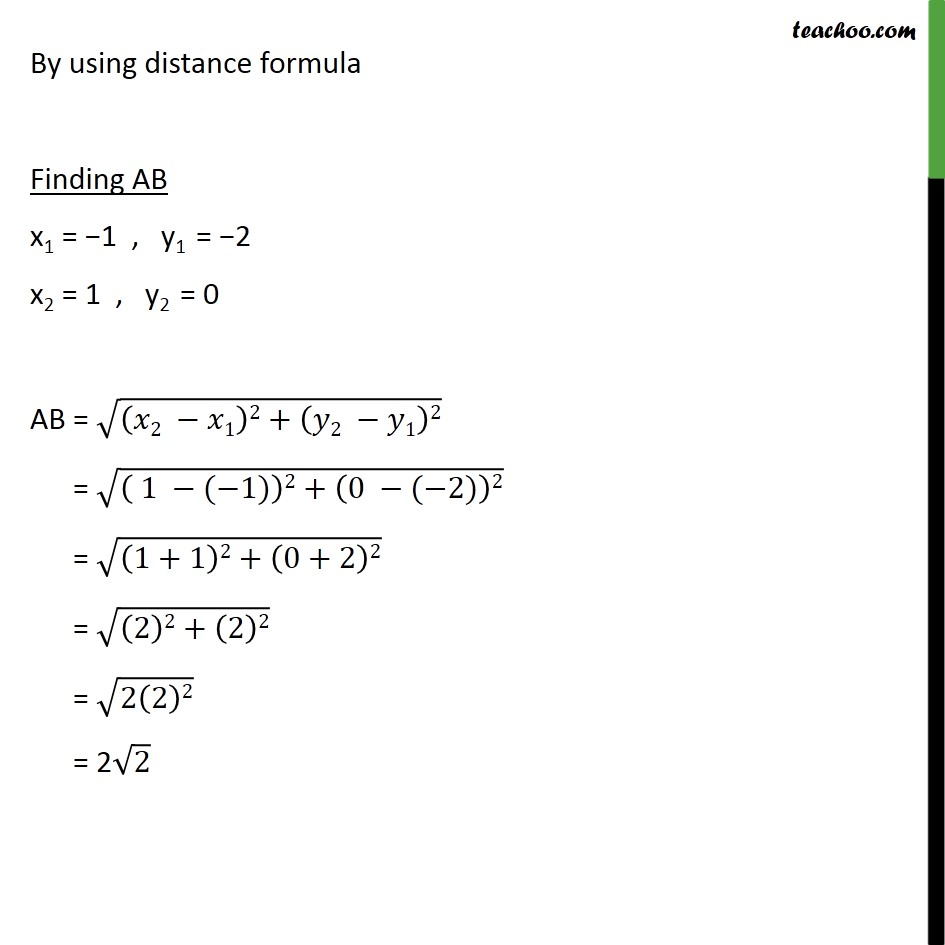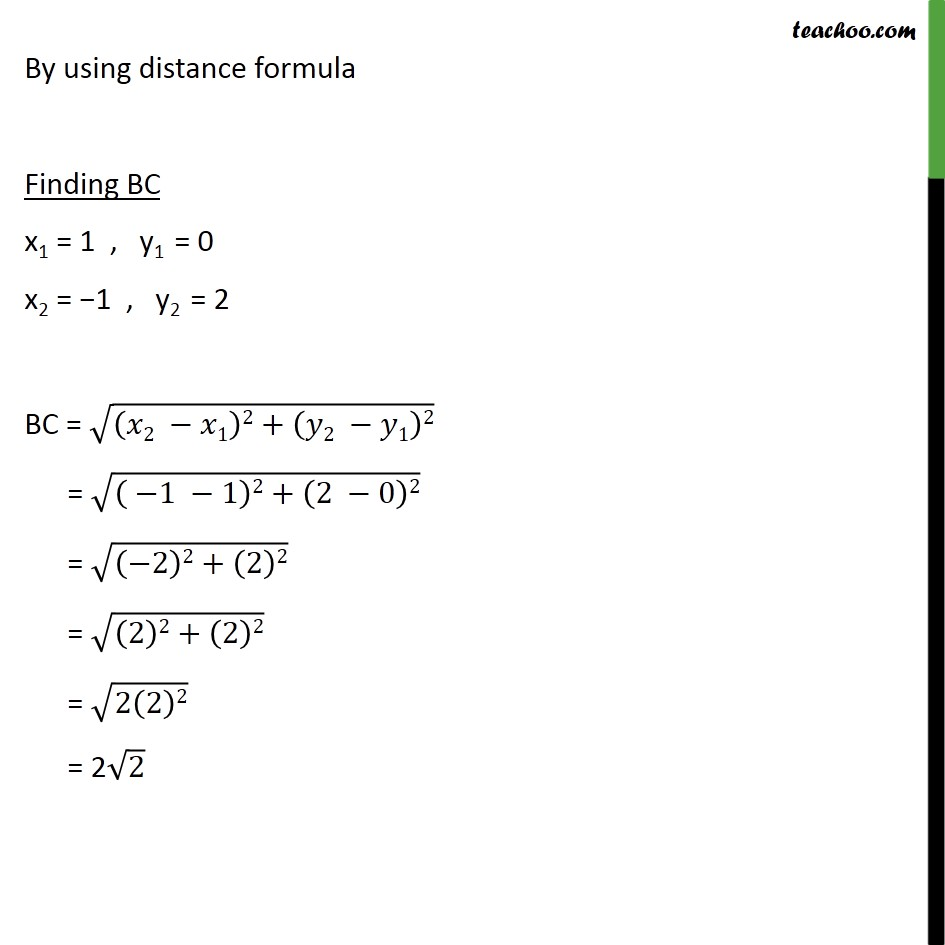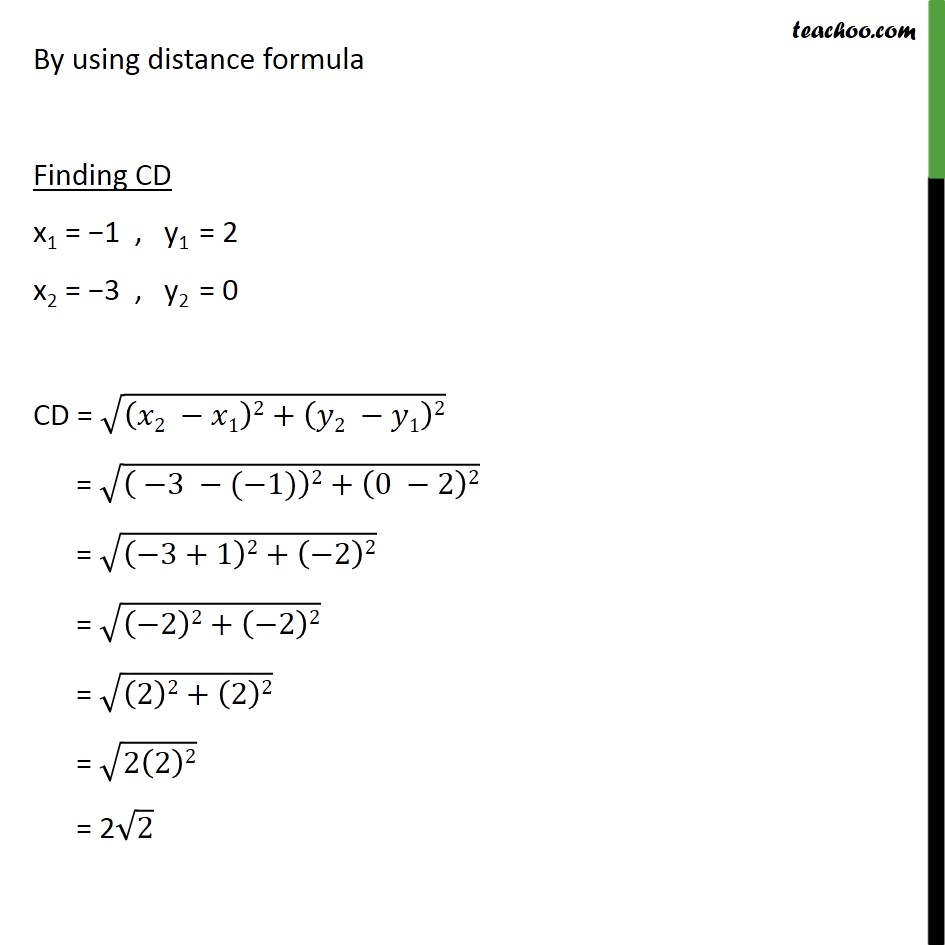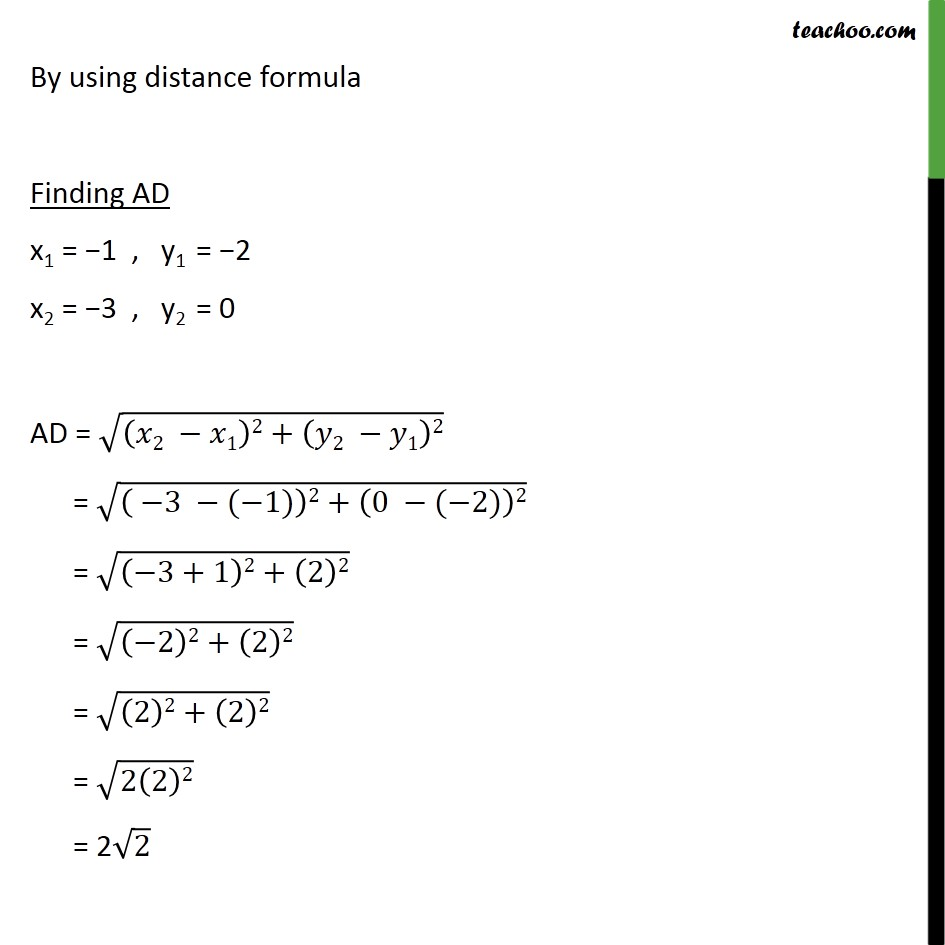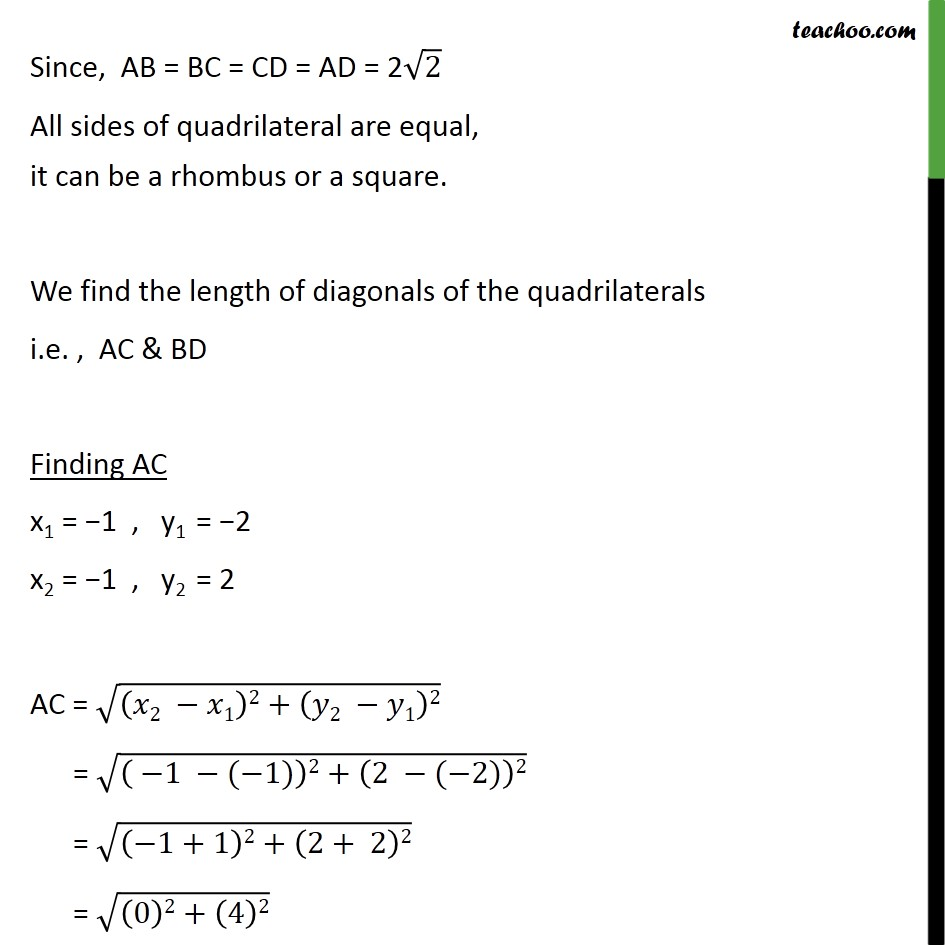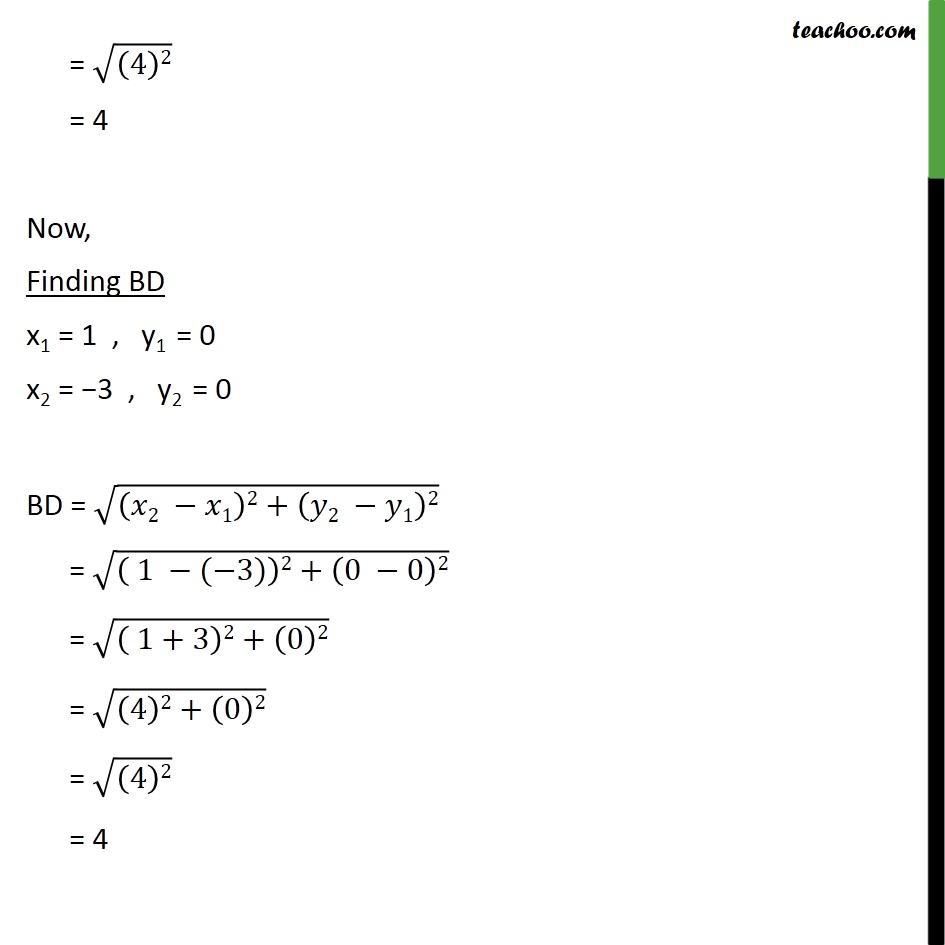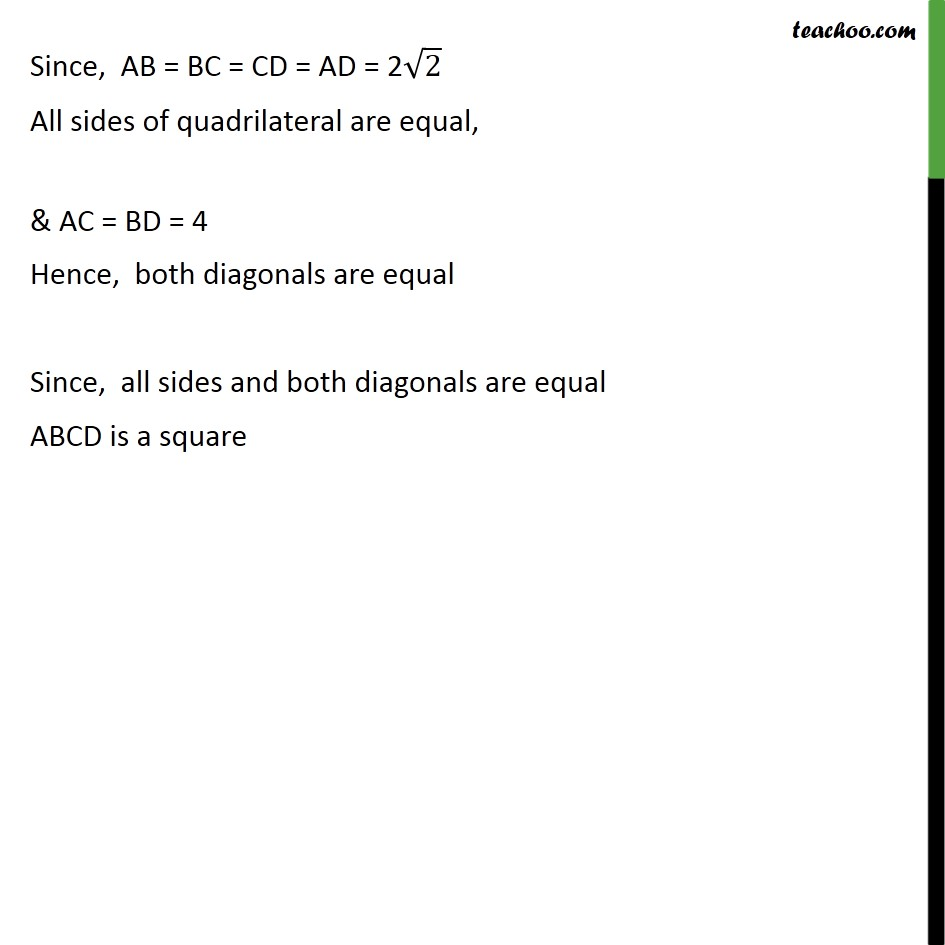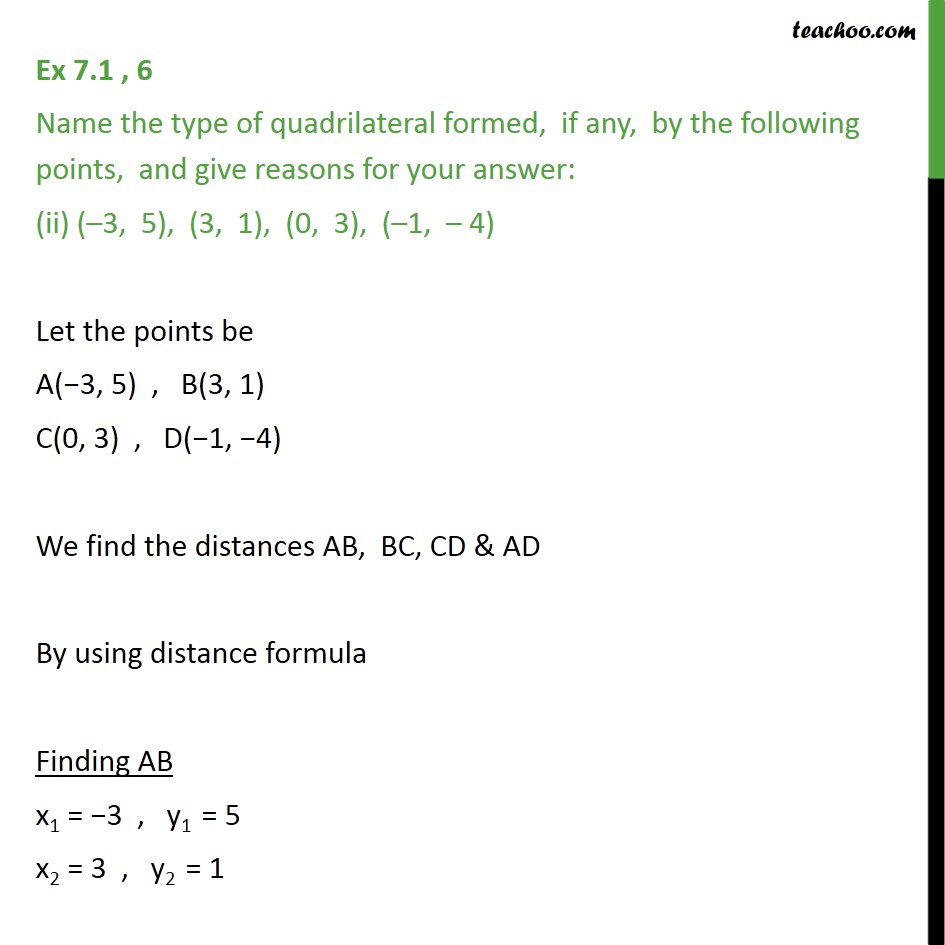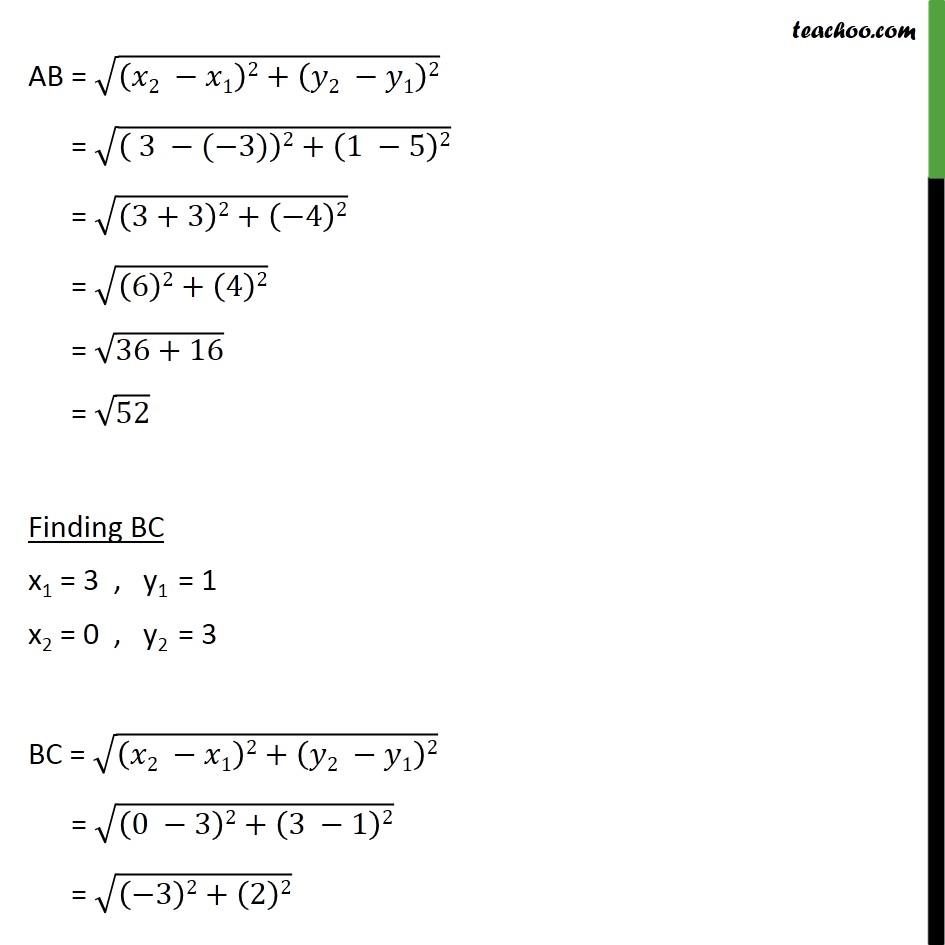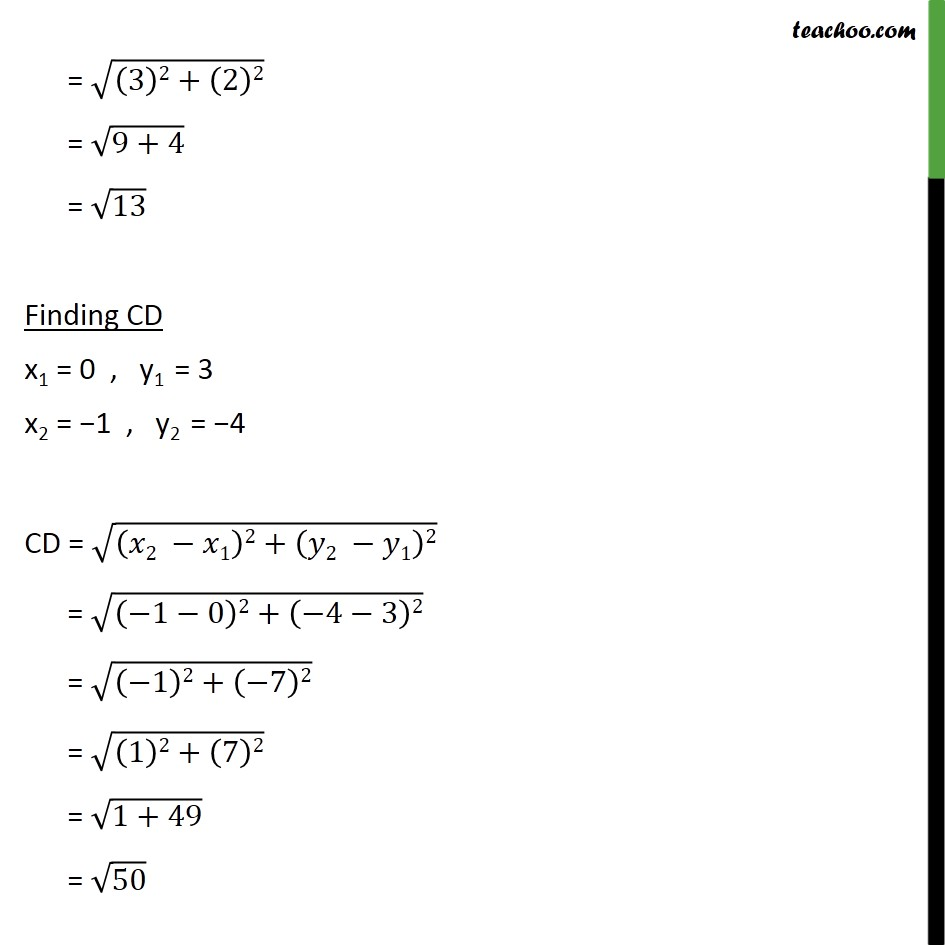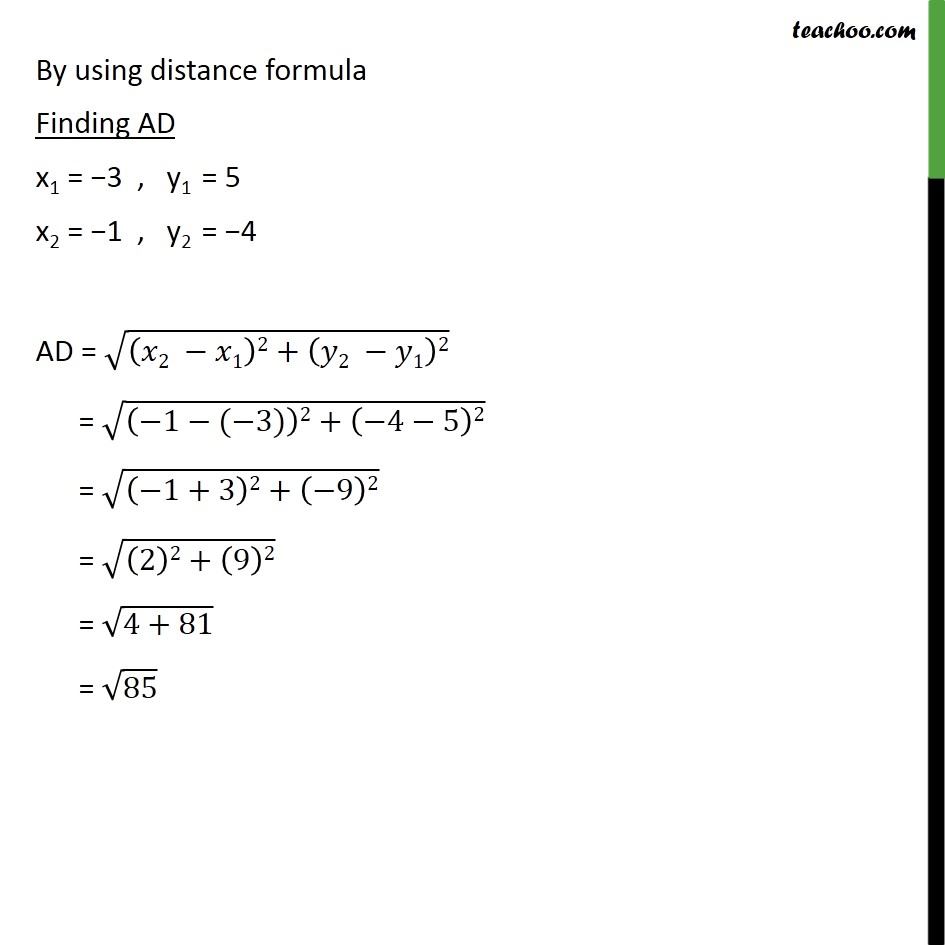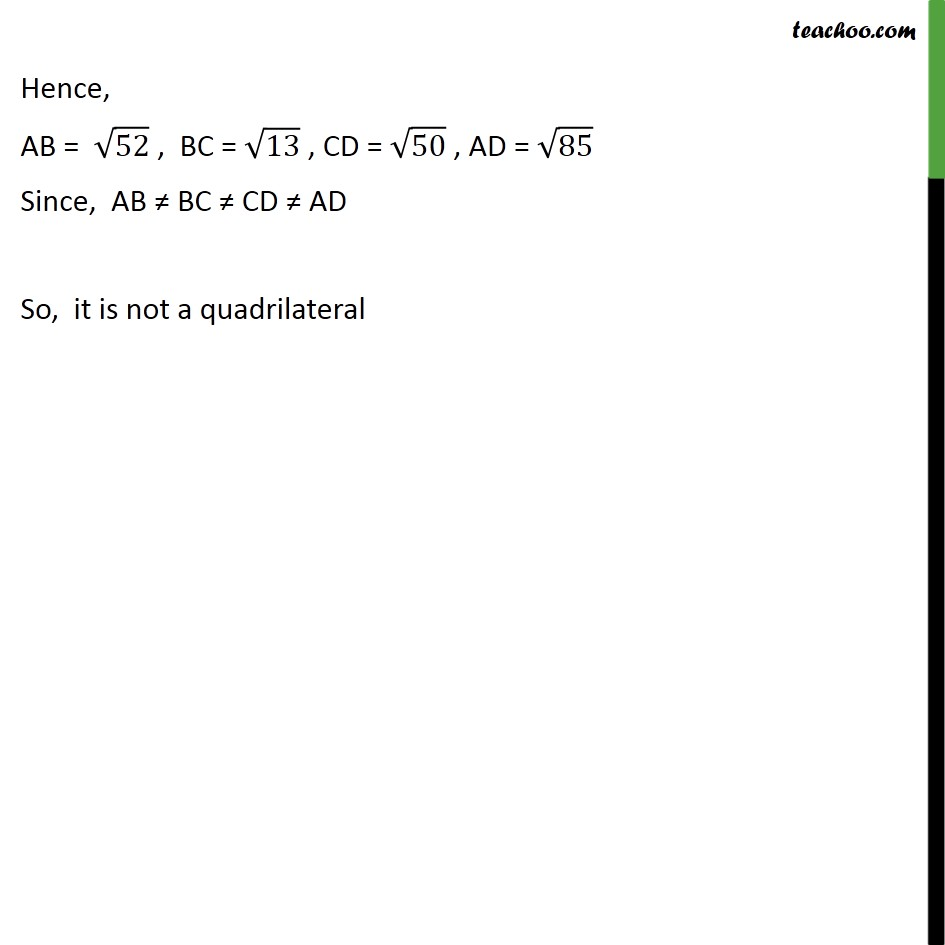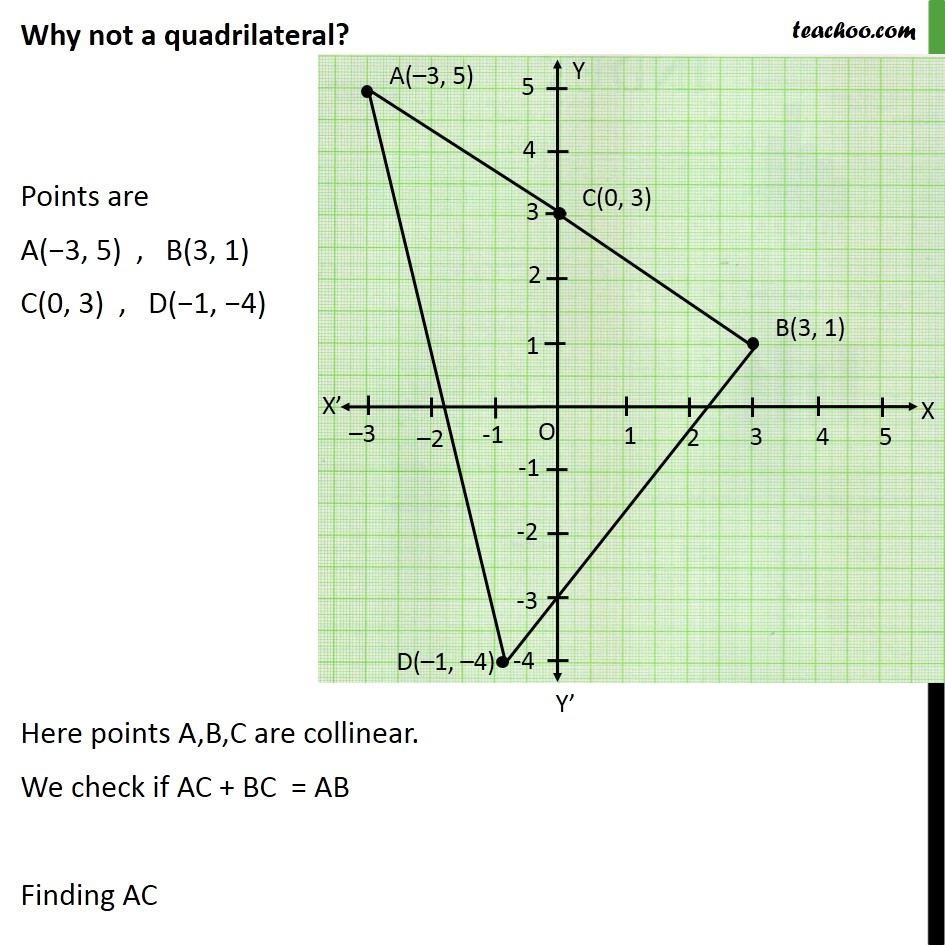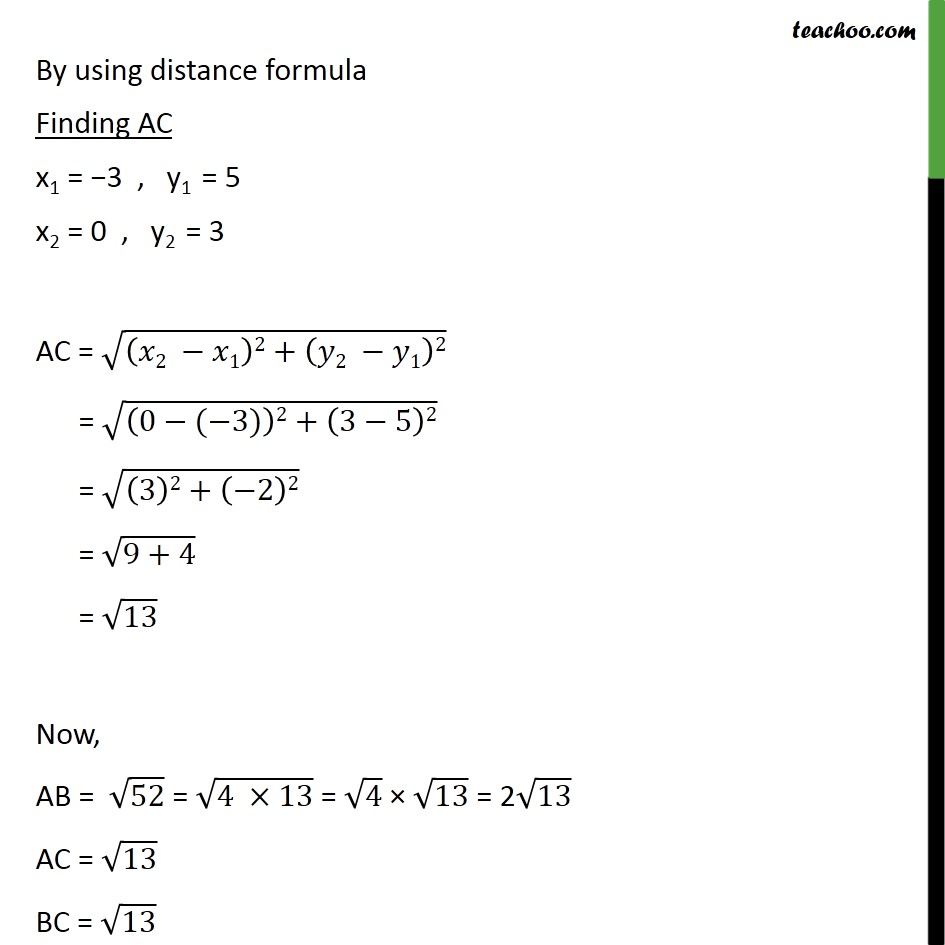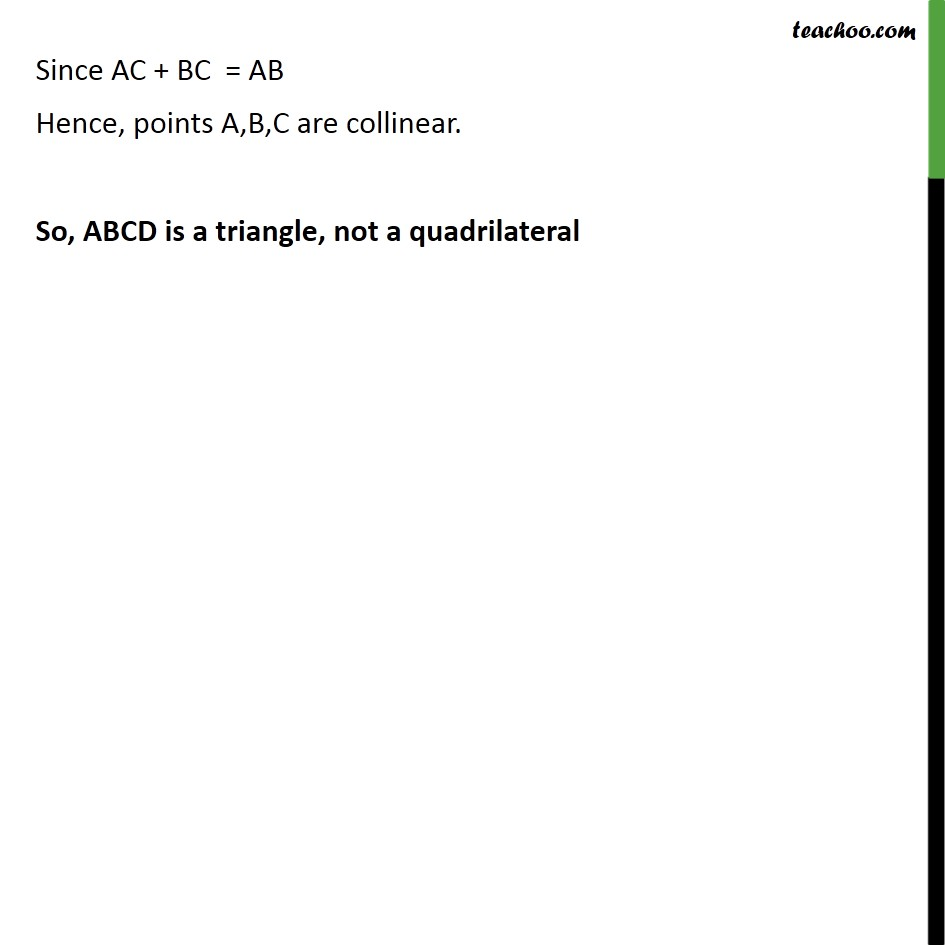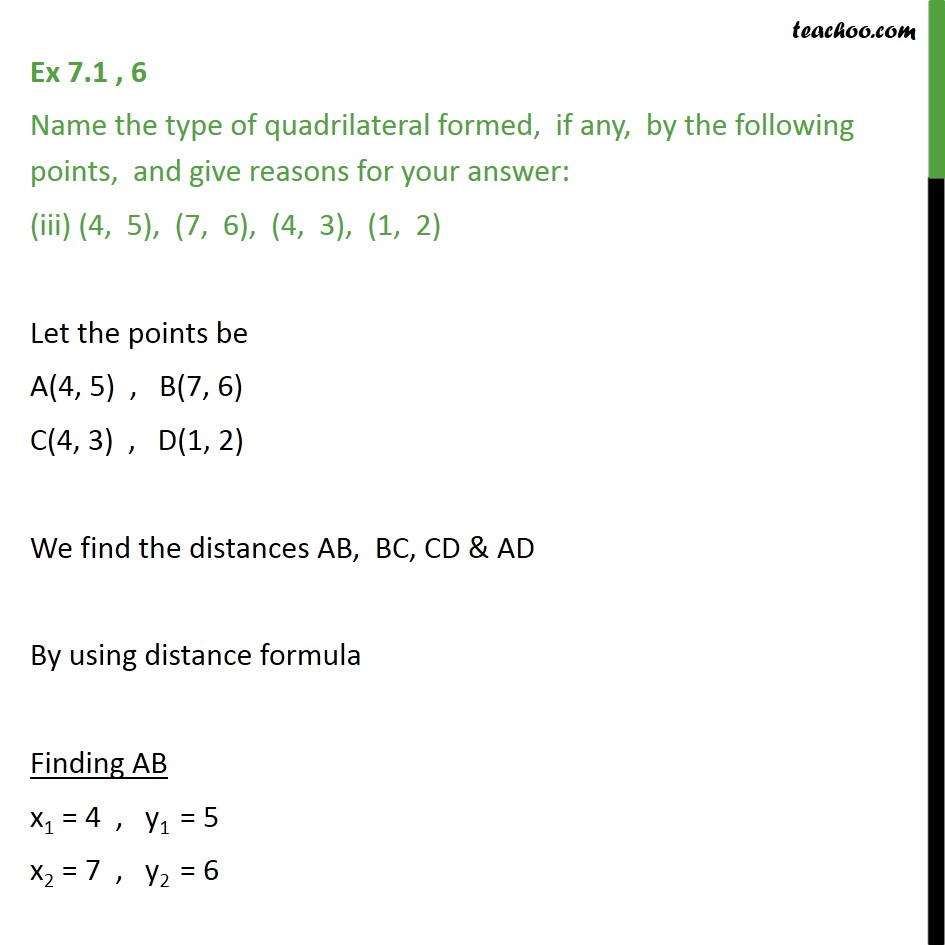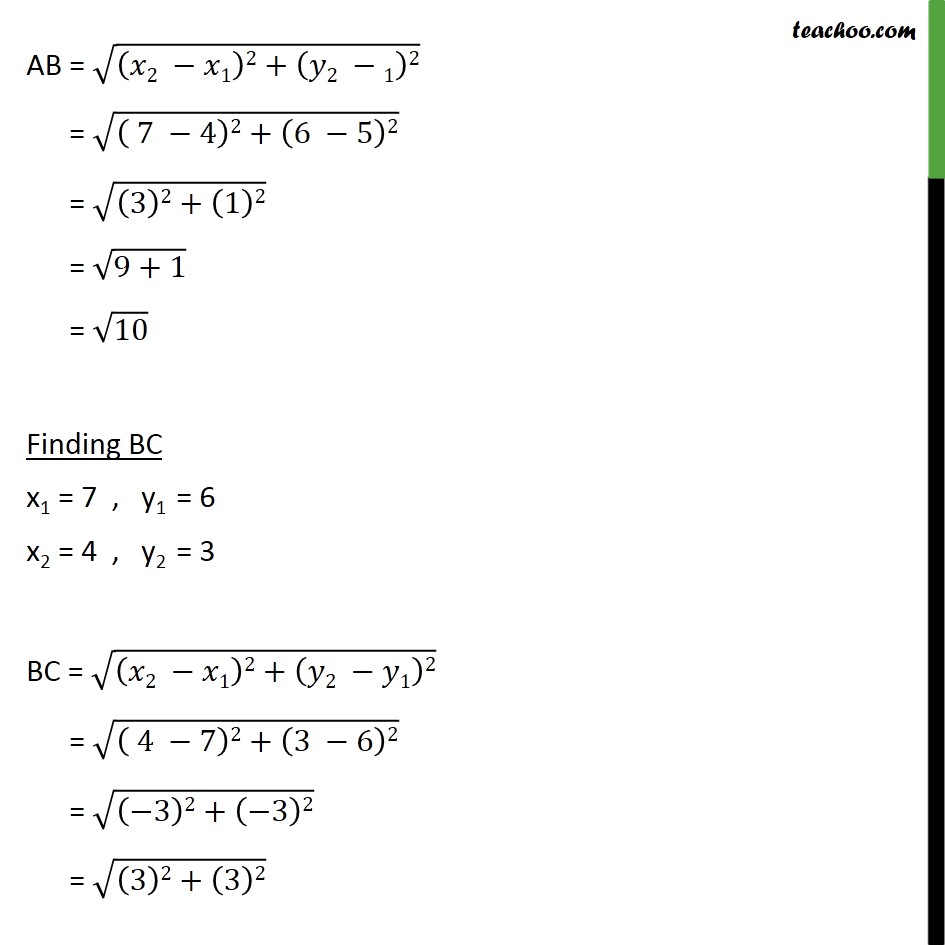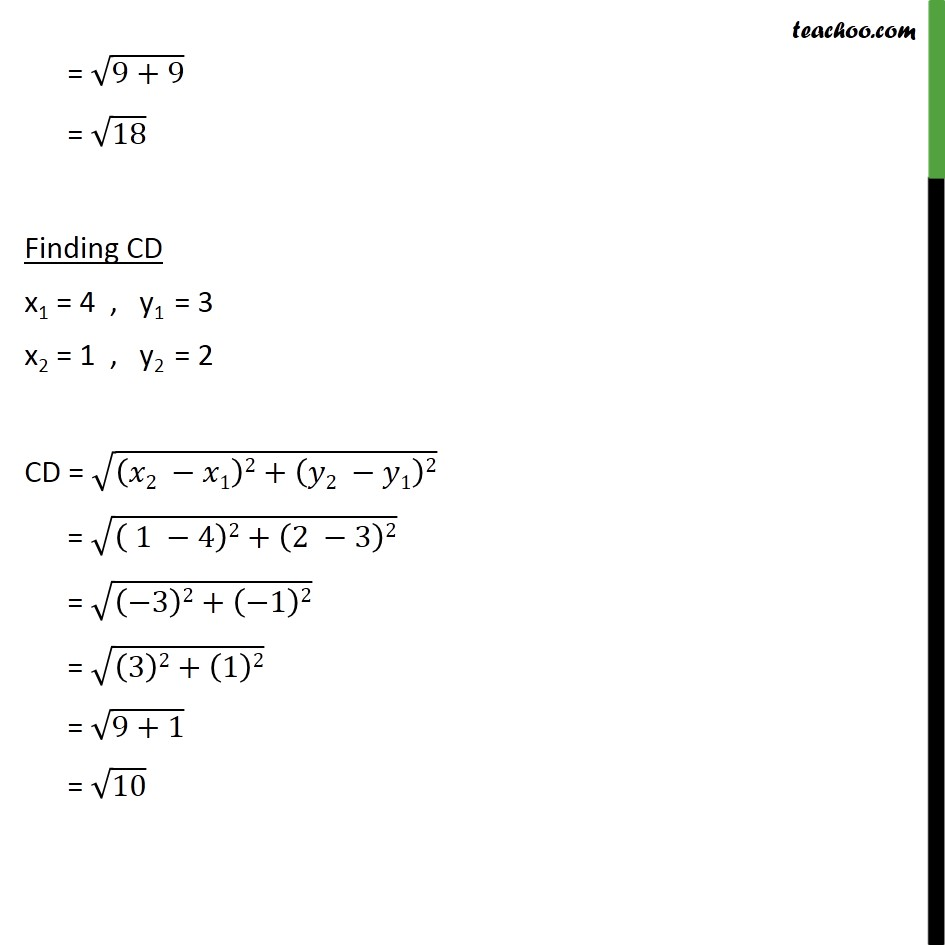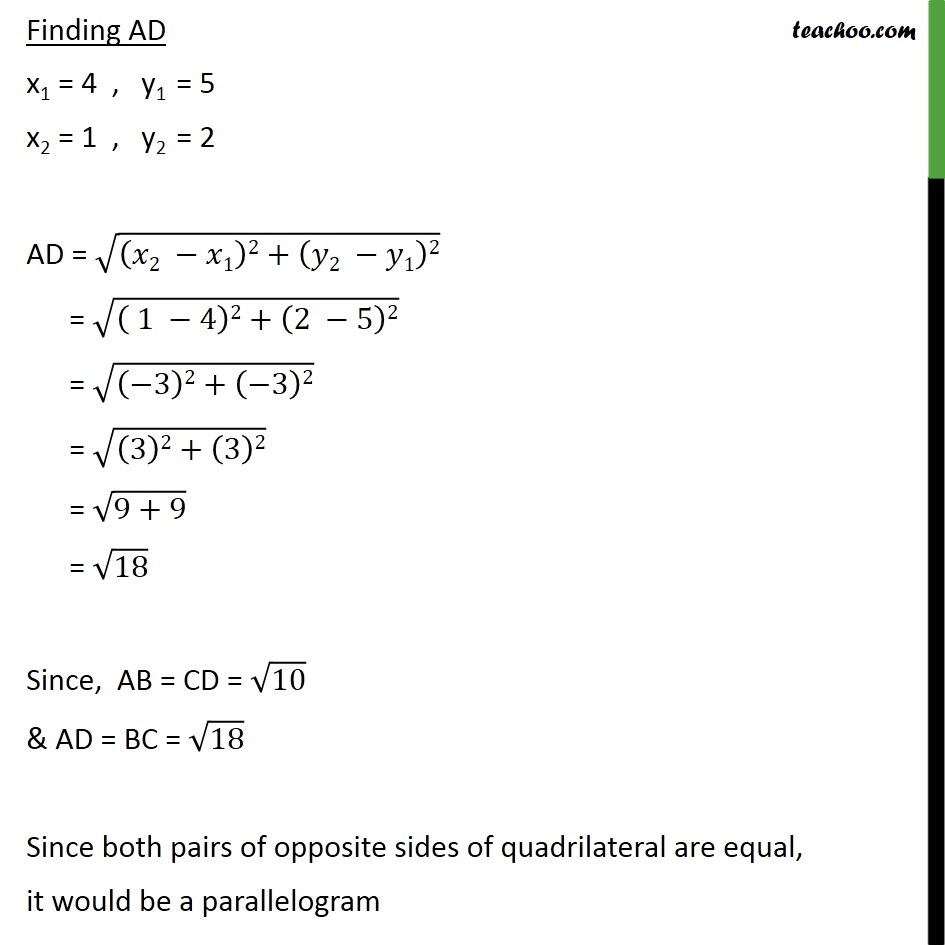1. Chapter 7 Class 10 Coordinate Geometry
2. Serial order wise
3. Ex 7.1

Transcript

Ex 7.1 , 6 Name the type of quadrilateral formed, if any, by the following points, and give reasons for your answer: (i) ( 1, 2), (1, 0), ( 1, 2), ( 3, 0) Let the points be A( 1, 2) , B(1, 0) C( 1, 2) , D( 3, 0) We find the distances AB, BC, CD & AD By using distance formula Finding AB x1 = 1 , y1 = 2 x2 = 1 , y2 = 0 AB = (( 2 1)2+( 2 1)2) = (( 1 ( 1))2+(0 ( 2))2) = ((1+1)2+(0+2)2) = ((2)2+(2)2) = (2(2)2) = 2 2 By using distance formula Finding BC x1 = 1 , y1 = 0 x2 = 1 , y2 = 2 BC = (( 2 1)2+( 2 1)2) = (( 1 1)2+(2 0)2) = (( 2)2+(2)2) = ((2)2+(2)2) = (2(2)2) = 2 2 By using distance formula Finding CD x1 = 1 , y1 = 2 x2 = 3 , y2 = 0 CD = (( 2 1)2+( 2 1)2) = (( 3 ( 1))2+(0 2)2) = (( 3+1)2+( 2)2) = (( 2)2+( 2)2) = ((2)2+(2)2) = (2(2)2) = 2 2 By using distance formula Finding AD x1 = 1 , y1 = 2 x2 = 3 , y2 = 0 AD = (( 2 1)2+( 2 1)2) = (( 3 ( 1))2+(0 ( 2))2) = (( 3+1)2+(2)2) = (( 2)2+(2)2) = ((2)2+(2)2) = (2(2)2) = 2 2 Since, AB = BC = CD = AD = 2 2 All sides of quadrilateral are equal, it can be a rhombus or a square. We find the length of diagonals of the quadrilaterals i.e. , AC & BD Finding AC x1 = 1 , y1 = 2 x2 = 1 , y2 = 2 AC = (( 2 1)2+( 2 1)2) = (( 1 ( 1))2+(2 ( 2))2) = (( 1+1)2+(2+ 2)2) = ((0)2+(4)2) = ((4)2) = 4 Now, Finding BD x1 = 1 , y1 = 0 x2 = 3 , y2 = 0 BD = (( 2 1)2+( 2 1)2) = (( 1 ( 3))2+(0 0)2) = (( 1+3)2+(0)2) = ((4)2+(0)2) = ((4)2) = 4 Since, AB = BC = CD = AD = 2 2 All sides of quadrilateral are equal, & AC = BD = 4 Hence, both diagonals are equal Since, all sides and both diagonals are equal ABCD is a square Ex 7.1 , 6 Name the type of quadrilateral formed, if any, by the following points, and give reasons for your answer: (ii) ( 3, 5), (3, 1), (0, 3), ( 1, 4) Let the points be A( 3, 5) , B(3, 1) C(0, 3) , D( 1, 4) We find the distances AB, BC, CD & AD By using distance formula Finding AB x1 = 3 , y1 = 5 x2 = 3 , y2 = 1 AB = (( 2 1)2+( 2 1)2) = (( 3 ( 3))2+(1 5)2) = ((3+3)2+( 4)2) = ((6)2+(4)2) = (36+16) = 52 Finding BC x1 = 3 , y1 = 1 x2 = 0 , y2 = 3 BC = (( 2 1)2+( 2 1)2) = ((0 3)2+(3 1)2) = (( 3)2+(2)2) = ((3)2+(2)2) = (9+4) = 13 Finding CD x1 = 0 , y1 = 3 x2 = 1 , y2 = 4 CD = (( 2 1)2+( 2 1)2) = (( 1 0)2+( 4 3)2) = (( 1)2+( 7)2) = ((1)2+(7)2) = (1+49) = 50 By using distance formula Finding AD x1 = 3 , y1 = 5 x2 = 1 , y2 = 4 AD = (( 2 1)2+( 2 1)2) = (( 1 ( 3))2+( 4 5)2) = (( 1+3)2+( 9)2) = ((2)2+(9)2) = (4+81) = 85 Hence, AB = 52 , BC = 13 , CD = 50 , AD = 85 Since, AB BC CD AD So, it is not a quadrilateral Why not a quadrilateral? Points are A( 3, 5) , B(3, 1) C(0, 3) , D( 1, 4) Here points A,B,C are collinear. We check if AC + BC = AB Finding AC By using distance formula Finding AC x1 = 3 , y1 = 5 x2 = 0 , y2 = 3 AC = (( 2 1)2+( 2 1)2) = ((0 ( 3))2+(3 5)2) = ((3)2+( 2)2) = (9+4) = 13 Now, AB = 52 = (4 13) = 4 13 = 2 13 AC = 13 BC = 13 Since AC + BC = AB Hence, points A,B,C are collinear. So, ABCD is a triangle, not a quadrilateral Ex 7.1 , 6 Name the type of quadrilateral formed, if any, by the following points, and give reasons for your answer: (iii) (4, 5), (7, 6), (4, 3), (1, 2) Let the points be A(4, 5) , B(7, 6) C(4, 3) , D(1, 2) We find the distances AB, BC, CD & AD By using distance formula Finding AB x1 = 4 , y1 = 5 x2 = 7 , y2 = 6 AB = (( 2 1)2+( 2 1)2) = (( 7 4)2+(6 5)2) = ((3)2+(1)2) = (9+1) = 10 Finding BC x1 = 7 , y1 = 6 x2 = 4 , y2 = 3 BC = (( 2 1)2+( 2 1)2) = (( 4 7)2+(3 6)2) = (( 3)2+( 3)2) = ((3)2+(3)2) = (9+9) = 18 Finding CD x1 = 4 , y1 = 3 x2 = 1 , y2 = 2 CD = (( 2 1)2+( 2 1)2) = (( 1 4)2+(2 3)2) = (( 3)2+( 1)2) = ((3)2+(1)2) = (9+1) = 10 Finding AD x1 = 4 , y1 = 5 x2 = 1 , y2 = 2 AD = (( 2 1)2+( 2 1)2) = (( 1 4)2+(2 5)2) = (( 3)2+( 3)2) = ((3)2+(3)2) = (9+9) = 18 Since, AB = CD = 10 & AD = BC = 18 Since both pairs of opposite sides of quadrilateral are equal, it would be a parallelogram

Ex 7.1

About the AuthorDavneet Singh
Davneet Singh is a graduate from Indian Institute of Technology, Kanpur. He has been teaching from the past 9 years. He provides courses for Maths and Science at Teachoo.